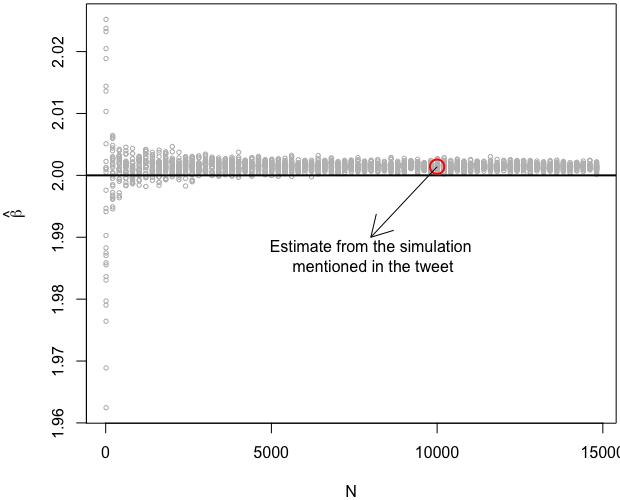# Simulating the OLS Consistency When X and ε are Dependent

## How to illustrate convergence in probability?

### Yihui Xie 2021-06-09

This morning I saw an interesting tweet by Nick HK, who ran a simulation to convince himself of the fact that OLS consistency only requires Corr(X, ε) = 0 but not independence:

I still find it hard to believe that OLS consistency only relies on X being uncorrelated with ε, not independent of ε, and I occasionally run a simulation to re-convince myself.I had no doubt about the conclusion, primarily because I had kindly returned most of the things I learned in the linear models class to the instructor after I passed the exams more than a decade ago (sorry, Dr. Nettleton!).

What I found not quite convincing was that Nick only ran the simulation once. A single estimate of $\beta$ is not convincing to me to show that the OLS estimator is consistent, even if its value (2.0014) is fairly close to the true parameter (2).

BTW, this is off-topic, but usually I’m slightly less convinced about simulation results obtained from a fixed random seed. Fixing the seed is common practice for reproducibility, but reproducibility should be independent with the seed (for random simulations, I think reproducibility “in the ballpark” is more useful than “digit-to-digit” reproducibility).

## A silly counterexample

If we could conclude consistency from an estimate in one simulation, we would conclude the same thing by estimating $\beta$ from the equation y = 2 * x + e, where e = 0.0014 * x, which means ε and X are perfectly correlated:

x = runif(10000)
e = .0014 * x
y = 2 * x + e  # i.e., y = 2.0014x
lm(y ~ x)


Apparently it would not be sensible, even if we get the same estimated value (2.0014), to conclude that consistency still exists when Corr(X, ε) = 1.

## N needs to increase to illustrate consistency

If I remember correctly, consistency means convergence (of $\hat{\beta}$ to $\beta$) in probability, as the sample size N increases to infinity. That means we need to examine the behavior of the estimator as N increases. Below is a function to estimate the slope of the simple linear regression, with a slight modification (changing lm() to lm.fit() for speed) based on Nick’s R code:

beta_est = function(N = 10) {
e = runif(N)
x = (e - .5) + 100 * (e - .5)^2 + rnorm(N)
y = 2 * x + e
res = lm.fit(cbind(1, x), y)
res$coefficients # the slope }  Next we estimate the coefficient 30 times for different sample sizes N = 10, 210, 410, …. Ns = rep(seq(10, 15000, 200), each = 30) bs = numeric(length(Ns)) for (i in seq_along(Ns)) { bs[i] = beta_est(Ns[i]) }  Last we draw a scatterplot to illustrate how the estimate “converges” to its true value. Note that is is only an illustration of the overall trend. Of course, an illustration is not a proof. par(mar = c(4, 4.5, .2, .2)) plot( Ns, bs, cex = .6, col = 'gray', xlab = 'N', ylab = expression(hat(beta)) ) abline(h = 2, lwd = 2) points(10000, 2.0014, col = 'red', cex = 2, lwd = 2) arrows(10000, 2.0014, 8000, 1.99, ) text(8000, 1.987, 'Estimate from the simulation mentioned in the tweet')I have also marked the value 2.0014 in the plot corresponding to the sample size 10000. ## Does it really converge to the true parameter value? At the first glance, the estimator appears to be converging indeed. However, after I marked the true parameter value (again, 2) with a horizontal line in the plot, it seems that it is converging to a value slightly higher than 2. I have to remind myself often that seeing is not believing. Instead, seeing can be a good start of thinking. Why does not the estimator appear to converge to 2? I do not know. I do not even know if I’m talking nonsense in this post (so now you know that I’m no longer qualified to be called Dr. Xie). My intuition is that $y \approx 2x + a\sqrt{x} + c$, where $a$ is a small constant. I will appreciate it if anyone could give a rigorous explanation. Update on 2021/06/10: Nick has given an explanation, which is quite obvious in hindsight. X and e are actually correlated in the above simulation, because X contains a linear term e, i.e., for X = e + 100e^2 + Z, e^2 and Z are not correlated with e, but e itself is certainly correlated with e. The correct simulation should be done without the linear term in X (or use abs(e), which is also not correlated with e): beta_est = function(N = 10) { e = runif(N) x = 100 * (e - .5)^2 + rnorm(N) y = 2 * x + e res = lm.fit(cbind(1, x), y) res$coefficients  # the slope
}